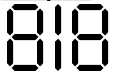# Check if mirror image of a number is same if displayed in seven segment displays in Python

Suppose we have a number n. We have to check whether the mirror image of the number is same as the given number or not when it is displayed on Seven Segment display.

So, if the input is like n = 818, then the output will be True.the mirror image is same.

To solve this, we will follow these steps −

• num_str := n as string
• for i in range 0 to size of num_str - 1, do
• if num_str[i] is not nay of ['0', '1', '8'] then, then
• return False
• left := 0
• right := size of num_str - 1
• while left < right, do
• if num_str[left] is not same as num_str[right], then
• return False
• left := left + 1
• right := right - 1
• return True

## Example

Let us see the following implementation to get better understanding −

Live Demo

def solve(n):
num_str = str(n)
for i in range(len(num_str)):
if num_str[i] not in ['0', '1', '8']:
return False
left = 0
right = len(num_str) - 1
while left < right:
if num_str[left] != num_str[right]:
return False
left += 1
right -= 1
return True
n = 818
print(solve(n))

## Input

818

## Output

True